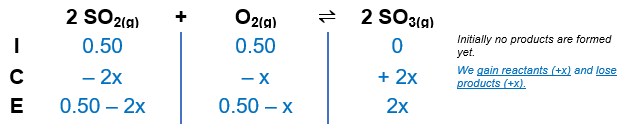# Problem: At 1100 K, Kp = 0.25 for the reaction2SO2(g) + O2(g) ⇌ 2SO3(g)Calculate the equilibrium partial pressures of SO2, O2, and SO3 produced from an initial mixture in which PSO2 = PO2 = 0.50 atm and PSO3 = 0. (Hint: If you don’t have a graphing calculator, then use the method of successive approximations to solve, as discussed in Appendix 1.4.)

###### FREE Expert Solution

2 SO2(g) + O2(g) ⇌ 2SO3(g)Kp = 0.25

Step 1Construct an ICE table.84% (17 ratings)###### Problem Details

At 1100 K, Kp = 0.25 for the reaction

2SO2(g) + O2(g) ⇌ 2SO3(g)

Calculate the equilibrium partial pressures of SO2, O2, and SO3 produced from an initial mixture in which PSO2 = PO2 = 0.50 atm and PSO3 = 0. (Hint: If you don’t have a graphing calculator, then use the method of successive approximations to solve, as discussed in Appendix 1.4.)